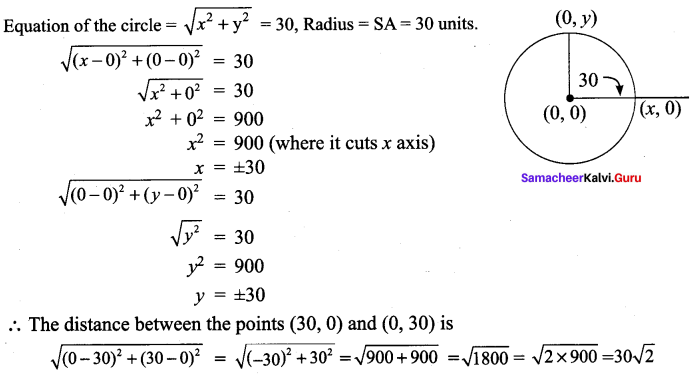# Samacheer Kalvi 9th Maths Solutions Chapter 5 Coordinate Geometry Ex 5.2

## Tamilnadu Samacheer Kalvi 9th Maths Solutions Chapter 5 Coordinate Geometry Ex 5.2

9th Maths Coordinate Geometry Exercise 5.2 Question 1.
Find the distance between the following pairs of points.
(i) (1, 2) and (4, 3)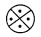(ii) (3, 4) and (- 7, 2)
(ii) (a, b) and (c, b)
(iv) (3, -9) and (-2, 3)
Solution:
We know that distance,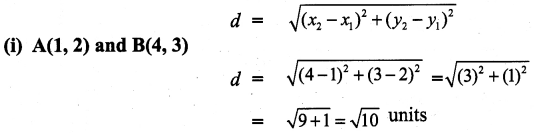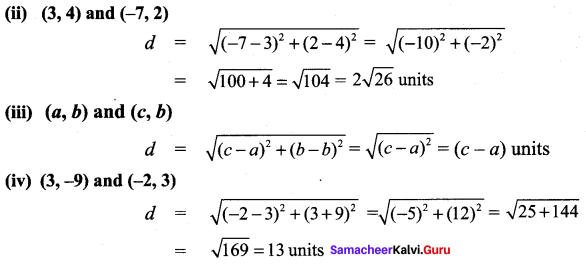9th Samacheer Maths Solution Ex 5.2 Question 2.
Determine whether the given set of points in each case are collinear or not.
(i) (7, -2), (5, 1), (3, 4)
(ii) (a, -2), (a, 3), (a, 0)
Solution:
(i) Let the points be A (7, -2), B (5, 1) and C (3, 4). By the distance formula.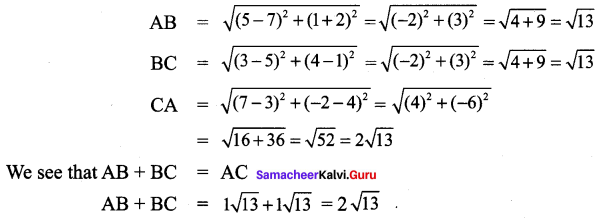∴ Hence the points are collinear.

(ii) Let the points be A (a, -2), B (a, 3) and C (a, 0).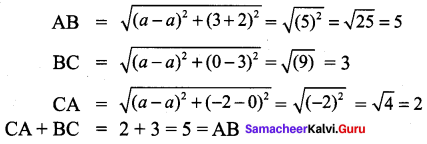∴ Hence the points are collinear.

Chapter 5 Coordinate Geometry Answers Question 3.
Show that the following points taken in order form an isosceles triangle.
(i) A (5, 4), B(2, 0), C (-2, 3)
(ii) A (6, -4),B (-2, -4), C (2, 10)
Solution:
(i) Let the points be A (5, 4), B (2, 0) and C (-2, 3)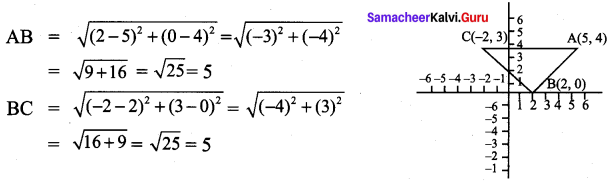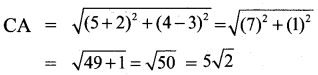Here AB + BC > CA and AB = BC. ∴ ∆ ABC is an isosceles triangle.

(ii) Let the points be A (6, -4), B (-2, -4) and C (2, 10).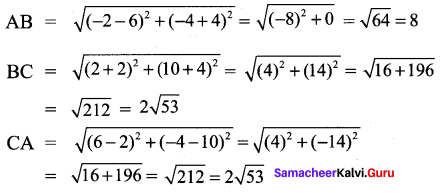Here BC + BA > CA and BC = CA. Two sides are equal, so ∆ ABC is an isosceles triangle

Coordinate Geometry Solutions Ex 5.2 Question 4.
Show that the following points taken in order form an equilateral triangle in each case.
(i) A(2, 2), B(-2, -2), C($$-2 \sqrt{3}$$, $$2 \sqrt{3}$$)
(ii) A($$\sqrt{3}$$ ,2), B (0, 1), C(0, 3)
Solution:
(i) Let the points be A (2, 2) B (-2, -2) and C($$-2 \sqrt{3}$$, $$2 \sqrt{3}$$)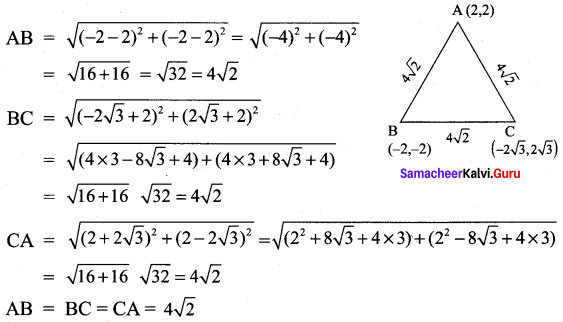All the 3 sides of ∆ABC are equal, Hence ∆ABC is an equilateral triangle.

(ii) Let the points be A ($$\sqrt{3}$$, 2), B (0, 1) and C (0, 3).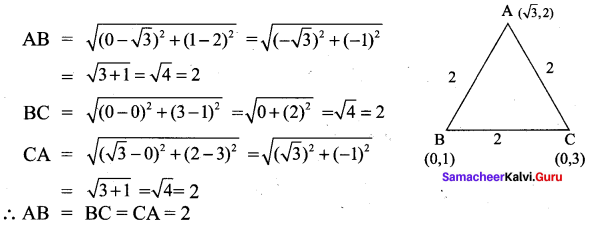All the 3 sides of ∆ABC are equal. Hence ∆ABC is an equilateral triangle.

Class 9 Maths Exercise 5.2 Solution Question 5.
Show that the following points taken in order form the vertices of a parallelogram.
(i) A(-3, 1), B(-6, -7), C (3, -9) and D(6, -1)
(ii) A (-7, -3), B(5, 10), C(15, 8) and D(3, -5)
Solution:
(i) Let A, B, C and D represent the points (-3, 1), (-6, -7) (3, -9) and (6, -1) respectively.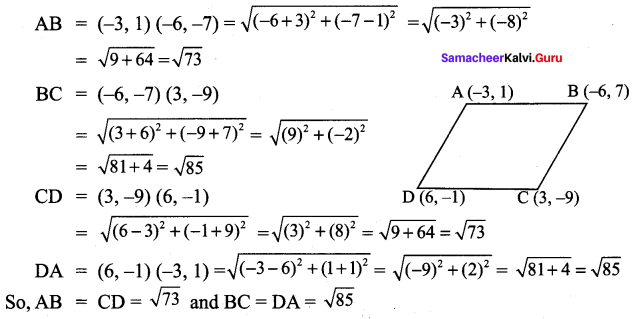The opposite sides are equal. Hence ABCD is a parallelogram.

(ii) Let A, B, C and D represent the points (-7, -3), (5, 10) (15, 8) and (3, -5)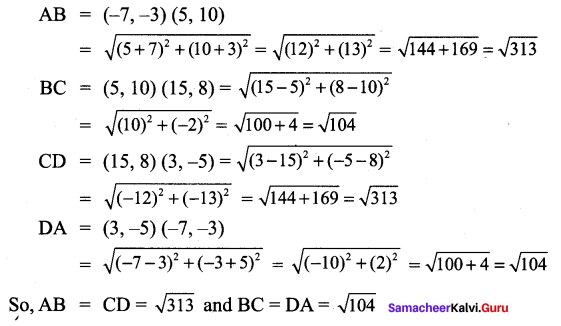The opposite sides are equal. Hence ABCD is a parallelogram.

Exercise 5.2 Class 9 Question 6.
Verify that the following points taken in order form the vertices of a rhombus.
(i) A(3, -2), B (7, 6),C (-1, 2) and D (-5, -6)
(ii) A (1, 1), B(2, 1),C (2, 2) and D(1, 2)
Solution:
(i) Let the points be A(3, -2), B (7, 6), C (-1, 2) and D (-5, -6)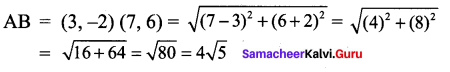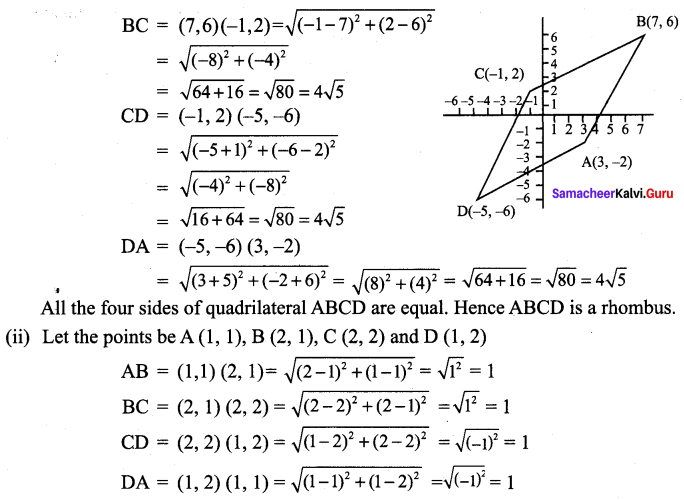∴ All the four sides of quadrilateral ABCD are equal. Hence ABCD is a rhombus.

9th Maths 5.2 Question 7.
If A(-1, 1), B(1, 3) and C(3, a) are points and if AB = BC, then find ‘a’
Solution: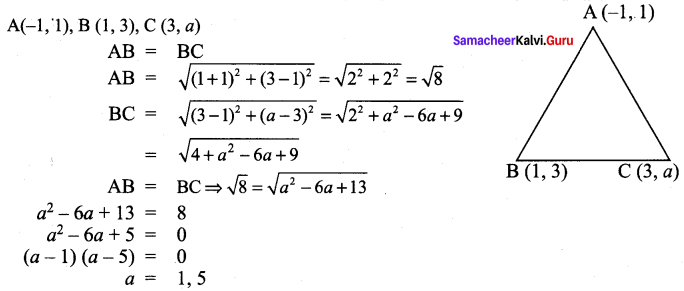Maths Solutions For Class 9 Samacheer Kalvi Ex 5.2 Question 8.
The abscissa of a point A is equal to its ordinate, and its distance from the point B(1, 3) is 10 units, what are the coordinates of A?
Solution: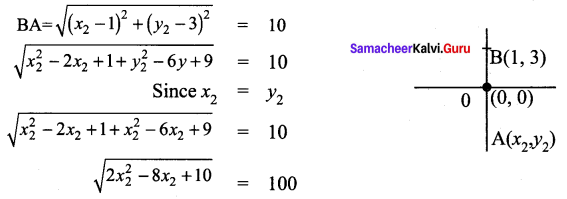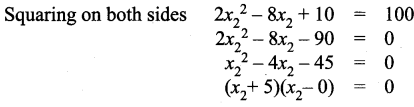Co-ordinates of A are (-5, -5) or (9, 9)

9th Maths Book Ex 5.2 Question 9.
The point (x, y) is equidistant from the points (3, 4) and (-5, 6). Find a relation between x and y.
Solution:
P(x, y) is equidistant from the points A(3, 4) and B(-5, 6)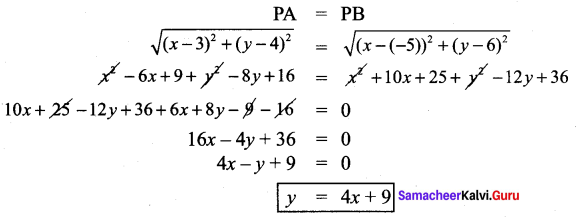Geometry 5.2  9th Maths Ex 5.2 Question 10.
Let A(2, 3) and B(2, -4) be two points. If P lies on the x-axis, such that AP = $$\frac{3}{7}$$AB, find the coordinates of P.
Solution: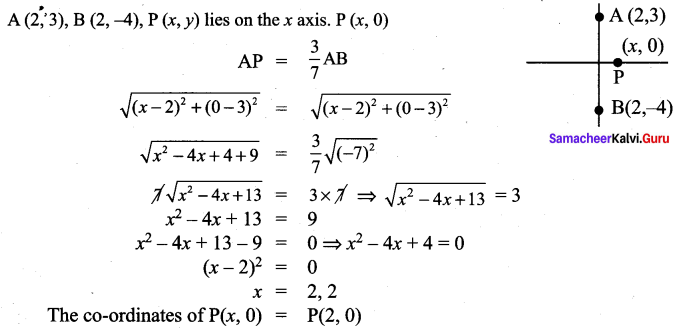9th Maths Guide Ex 5.2 Question 11.
Show that the point (11, 2) is the centre of the circle passing through the points (1, 2), (3, -4) and (5, -6)
Solution: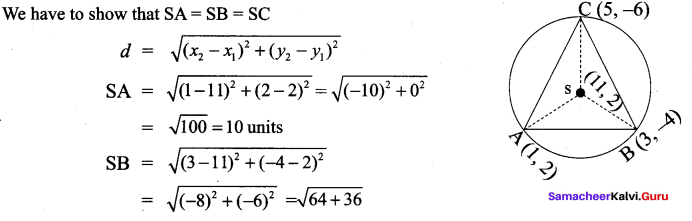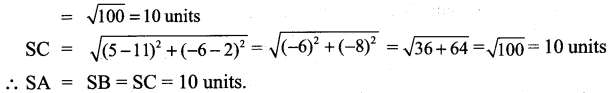Therefore S is the centre of the circle, passing through A, B and C.

5 Coordinate Geometry Ex 5.2 Question 12.
The radius of a circle with centre at origin is 30 units. Write the coordinates of the points where the circle intersects the axes. Find the distance between any two such points.
Solution: